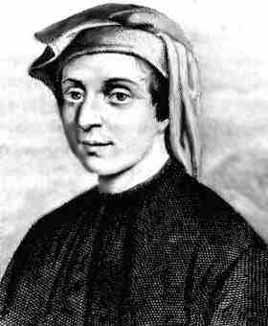## Playing with 56

Find the smallest number n such that the digit sum of n is equal to 56, the number n is divisible by 56 and its two last digits are Read more..

## Fibonacci Sequence: A Pattern of Natural Beauty

Fibonacci sequence can be found everywhere in nature. First mentioned by Leonardo Fibonacci, a medieval mathematician, who was trying to solve the problem of rabbit population growth. It became one of the most famous sequence in all of mathematics, describing innumerous patterns of natural phenomena. Here is a mathematical description for some of its fundamental properties. Read more
Tags: Math## Why the graph of quadratic function is a parabola?

Using no more than Pythagorean theorem it can be shown that the graph of any quadratic function is a parabola Read more...

## How to use reverse and forward search with Vim and Zathura

Reverse and forward search are very helpful features in LaTex compilation. Here is how we do it with vim and zathura... read more

## Free textbooks in mathematics

The following is a list of links to useful textbooks in mathematics, available for free on the Internet. All books are legally safe to download, The books are in printable format - Postscript (PS) or Portable Document Format (PDF). You are free to download, read and print them. Read more...

## Math related Videos

YouTube channels, videos and course lectures.

terms